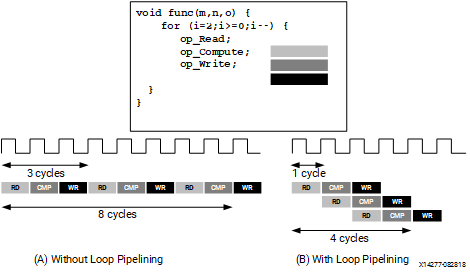# Loop Pipelining - 2022.1 English

## Vitis Unified Software Platform Documentation: Application Acceleration Development (UG1393)

Document ID
UG1393
Release Date
2022-05-25
Version
2022.1 English

By default, every iteration of a loop only starts when the previous iteration has finished. In the loop example below, a single iteration of the loop adds two variables and stores the result in a third variable. Assume that in hardware this loop takes three cycles to finish one iteration. Also, assume that the loop variable `len` is 20, that is, the `vadd` loop runs for 20 iterations in the kernel. Therefore, it requires a total of 60 clock cycles (20 iterations * 3 cycles) to complete all the operations of this loop.

``````vadd: for(int i = 0; i < len; i++) {
c[i] = a[i] + b[i];
}``````
Tip: It is good practice to always label a loop as shown in the above code example (`vadd:…`). This practice helps with debugging when working in the Vitis core development kit. Note that the labels generate warnings during compilation, which can be safely ignored.
Pipelining the loop executes subsequent iterations in a pipelined manner. This means that subsequent iterations of the loop overlap and run concurrently, executing at different sections of the loop-body. Pipelining a loop can be enabled by the . Note that the pragma is placed inside the body of the loop.
``````vadd: for(int i = 0; i < len; i++) {
#pragma HLS PIPELINE
c[i] = a[i] + b[i];
}``````

In the example above, it is assumed that every iteration of the loop takes three cycles: read, add, and write. Without pipelining, each successive iteration of the loop starts in every third cycle. With pipelining the loop can start subsequent iterations of the loop in fewer than three cycles, such as in every second cycle, or in every cycle.

The number of cycles it takes to start the next iteration of a loop is called the initiation interval (II) of the pipelined loop. So II = 2 means each successive iteration of the loop starts every two cycles. An II = 1 is the ideal case, where each iteration of the loop starts in the very next cycle. When you use `pragma HLS PIPELINE`, the compiler always tries to achieve II = 1 performance.

The following figure illustrates the difference in execution between pipelined and non-pipelined loops. In this figure, (A) shows the default sequential operation where there are three clock cycles between each input read (II = 3), and it requires eight clock cycles before the last output write is performed.

Figure 1. Loop PipeliningIn the pipelined version of the loop shown in (B), a new input sample is read every cycle (II = 1) and the final output is written after only four clock cycles: substantially improving both the II and latency while using the same hardware resources.
Important: Pipelining a loop causes any loops nested inside the pipelined loop to get unrolled.

If there are data dependencies inside a loop, as discussed in Loop Dependencies, it might not be possible to achieve II = 1, and a larger initiation interval might be the result.